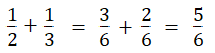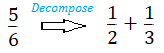# Method of Partial Fractions

## What is The Method of Partial Fractions?

The method of partial fractions decomposes (breaks apart) a rational function into a set of simpler rational functions. It’s based on the fact that you can add fractions with a common denominator. For example:We could reverse (“decompose”) this:You can also decompose a function the same way. For example:## Integrating a Decomposed Function

Once you’ve “decomposed” the fraction, you can integrate the separate parts. Using the above example, we know that the integral of 1/x is ln |x| + c, so:You could choose to find the integral without partial fractions, but it would be a fairly lengthy process involving completing the square (where you rewrite a polynomial as a quantity raised to the power of “2”) and trigonometric substitution. The method of partial fractions gives you a result with a tenth of the work.

## When to Use the Method of Partial Fractions

Partial fraction decomposition only works for proper rational functions—rational functions where the degree of the numerator is less than the degree of the denominator.

The method of partial fractions often makes it much easier—and faster—to find an integral, you do have to have some strong algebra skills to be able to find the partial fractions. Plus, the more integrals you know the better, so that you can recognize when it makes sense to decompose a fraction (and when it doesn’t). If algebra and top-of-your-head knowledge of integrals isn’t your forte, you might find it easier to look for alternatives like:

While the technique can be easy, finding the partial fractions in the first place can be an extreme challenge if your algebra is weak. There are several “rules” to get your fractions into the right form (see below). If the thought of rules give you an algebra headache, try the The Symbolab calculator, which gives step by step solutions for partial fractions.

## Rules to Find Partial Fractions

The decomposition will be a sum of terms where the numerators contain coefficients (A, B, or C). The number of the coefficient will equal the degree in the denominator.

1. Linear term, (ax + b) →2. Repeated linear term, e.g. (ax + b)33. Quadratic term ax2 + bx + c →4. Repeated quadratic term , e.g. (ax2 + bx + c)2